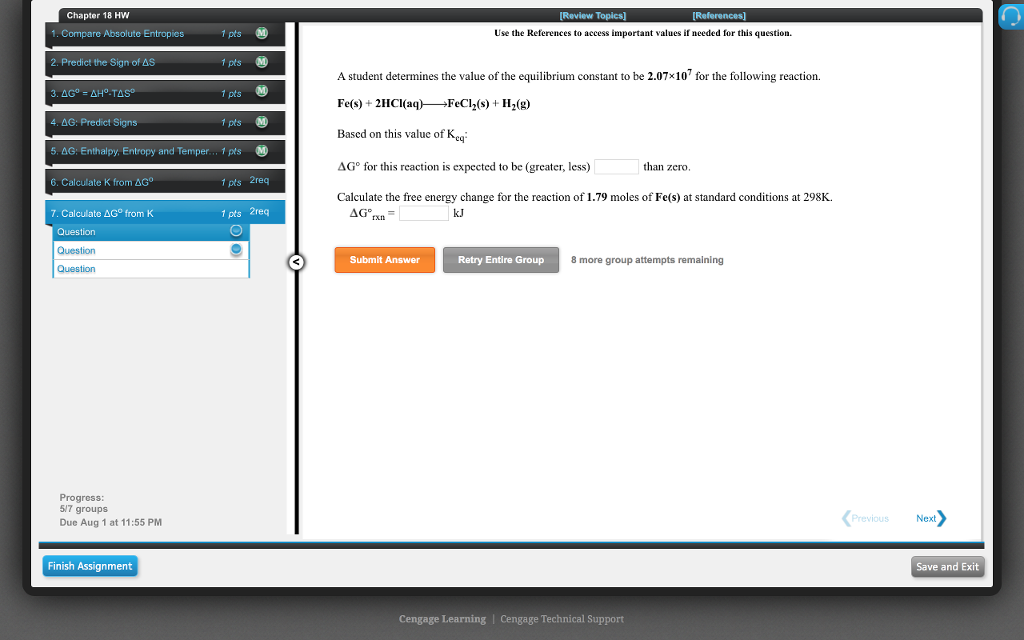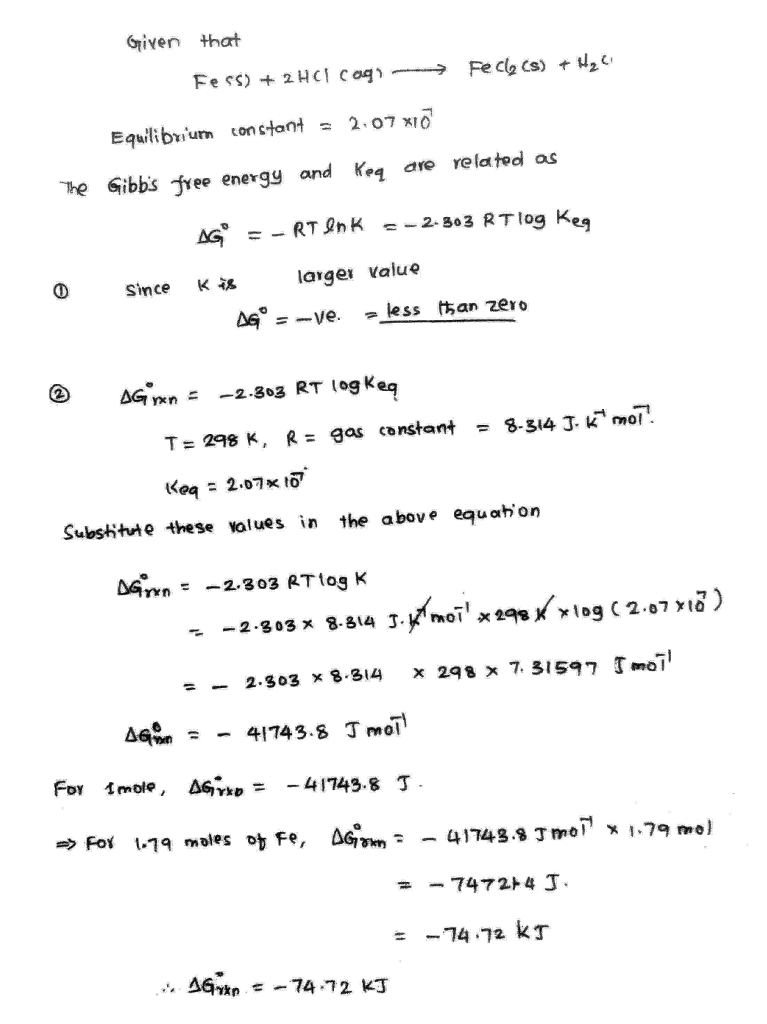# Question & Answer: A student determines the value of the equilibrium constant to be 2.07×107 for the following…..

A student determines the value of the equilibrium constant to be 2.07×107 for the following reaction.

Fe(s) + 2HCl(aq)—–>FeCl2(s) + H2(g)

Based on this value of Keq:G° for this reaction is expected to be (greater, less)____?____ than zero.

Calculate the free energy change for the reaction of 1.79 moles of Fe(s) at standard conditions at 298K.rxn =_____?_____ kJ